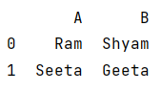# Appending a list or series to a pandas DataFrame as a row

Given a Pandas DataFrame, we have to append a list or series in it.
Submitted by Pranit Sharma, on June 20, 2022

Pandas is a special tool that allows us to perform complex manipulations of data effectively and efficiently. Inside pandas, we mostly deal with a dataset in the form of DataFrame. DataFrames are 2-dimensional data structures in pandas. DataFrames consist of rows, columns, and the data.

A list is a collection of the heterogeneous type of elements which means the data inside a list can be of any type. A list in python is just like an array where the first element has an index of 1 and the last element has an index n-1 where n is the length of the list.

A series is just like a list but the only difference is when we print a series it appears in a vertical fashion which represents that it consists of a single column and each element acts as a row.

To learn how to append a list or series to a pandas DataFrame as a row, we will first append a list inside a list and then convert it into a DataFrame.

To work with pandas, we need to import pandas package first, below is the syntax:

```import pandas as pd
```

Let us understand with the help of an example,

## Python code to append a list or series to a pandas DataFrame as a row

```# Importing pandas package
import pandas as pd

# Creating a list of list and appending another
# list inside it
list = [['Ram','Shyam']]
list.append(['Seeta','Geeta'])

# Adding these lists in df
df = pd.DataFrame(list,columns=['A','B'])

# Display DataFrame
print(df)
```

Output:Preparation

What's New

Top Interview Coding Problems/Challenges!